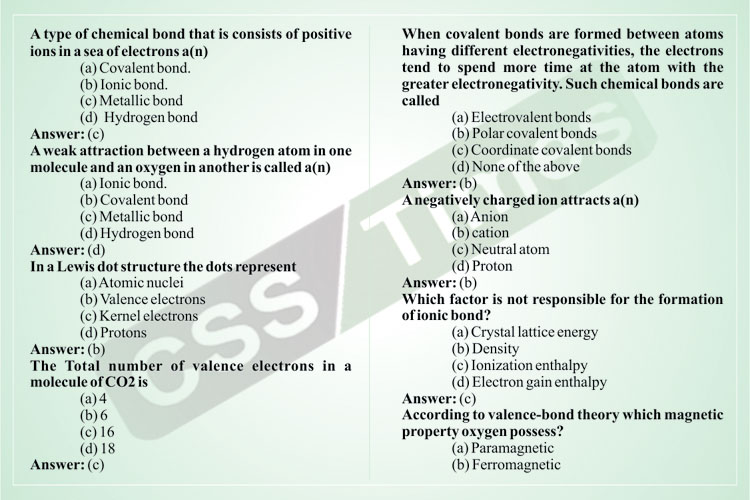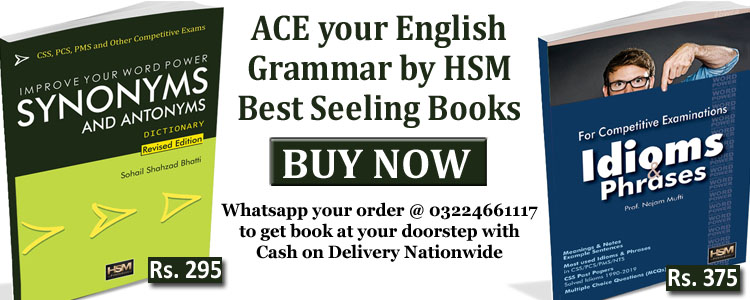# Atomic Structure MCQs (Set-II) | General Science and Ability Solved MCQsWritten by

A type of chemical bond that is consists of positive ions in a sea of electrons a(n)
(a) Covalent bond.
(b) Ionic bond.
(c) Metallic bond
(d) Hydrogen bond

A weak attraction between a hydrogen atom in one molecule and an oxygen in another is called a(n)
(a) Ionic bond.
(b) Covalent bond
(c) Metallic bond
(d) Hydrogen bond

In a Lewis dot structure the dots represent
(a) Atomic nuclei
(b) Valence electrons
(c) Kernel electrons
(d) Protons

#### Check Also: Industrial and Agricultural Revolution | Notes for CSS / PMS

The Total number of valence electrons in a molecule of CO2 is
(a) 4
(b) 6
(c) 16
(d) 18When covalent bonds are formed between atoms having different electronegativities, the electrons tend to spend more time at the atom with the greater electronegativity. Such chemical bonds are called
(a) Electrovalent bonds
(b) Polar covalent bonds
(c) Coordinate covalent bonds
(d) None of the above

A negatively charged ion attracts a(n)
(a) Anion
(b) cation
(c) Neutral atom
(d) Proton

Which factor is not responsible for the formation of ionic bond?
(a) Crystal lattice energy
(b) Density
(c) Ionization enthalpy
(d) Electron gain enthalpy

According to valence-bond theory which magnetic property oxygen possess?
(a) Paramagnetic
(b) Ferromagnetic
(c) Diamagnetic
(d) Anti Ferromagnetic

Who was proposed valence-bond theory?
(a) Mulliken
(b) Linus Pauling
(c) Heitler–London
(d) Hund

Which of the following sentence is incorrect for covalent bond?
(a) Strength of covalent bond depends upon overlapping at atomic orbitals.
(b) Covalent bond is not directional.
(c) There is sharing of electrons between atoms bonded by covalent bond
(d) Covalent bond is formed between atoms having less difference in their electronegativity.

Crystal formation is which type of reaction ?
(a) endothermic and exothermic
(b) endothermic
(c) exothermic
(d) no heat change occurs

Lattice energy of ionic compound depends upon which factor ?
(a) Size of ion
(b) Size of ion and charge
(c) charge on ion
(d) Arrangement of ion

#### Check Also: General Science and Ability MCQs Solved (Exercise-1)

Maximum how many numbers of hydrogen bond can be form by H2O molecule ?
(a) 2
(b) 4
(c) 3
(d) 1

On keeping two cube of ice on each other which become one cube which factor is responsible for it ?
(a) Van-Der Waals attraction
(b) Hydrogen bond
(c) Dipole attraction
(d) Covalent bond

Number of H – bond form by unpaired electrons of liquid NH3 ,H2O and HF respectively are
(a) 3, 4, 2
(b) 4, 4, 2
(c) 3, 2, 1
(d) 1, 2, 1

Which of the following characteristic does not possesses by metal?
(a) luminus
(b) ductility
(c) increase in conductance by increase in temperature
(d) malleability

On which factor conductance of metals responsible ?
(a) ions
(b) delocalized
(c) atomic kernel
(d) number of atoms

On which factor van-der waalls attraction force does not depend ?
(a) numbers of molecules
(b) contact surface area of molecules
(c) shape of molecules
(d) numbers of electron in molecules

Which rule is violated in the given electronic configuration ?
(a) Aufbau
(b) Pauli
(c) Hund
(d) Given all

Which theory is useful to determine geometrical structure of molecules ?
(a) molecular orbital theory
(b) VSEPR theory
(c) Resonance theory
(d) Quantum mechanics

The one outermost electron present in Na element at
(a) one corner of simple cube
(b) eight corner of simple cube
(c) center of simple cube
(d) each corner of simple cube

NH3 has a higher boiling point than expected because
(a) its density decreases on freezing
(b) with water it forms NH4OH
(c) it has strong inter molecular covalent bonds ?
(d) it has intermolecular hydrogen bonds.

The molecule with zero dipole moment is
(a) chloroform
(b) methyl chloride
(c) carbon tetrachloride
(d) methylene chloride

In OF2, number of bond pairs and lone pairs of electrons are respectively
(a) 2, 8
(b) 2, 6
(c) 2, 9
(d) 2, 10

Bond strength increases with
(a) Bond length increasing
(b) Anti bonding electrons being higher in number
(c) Bond order increasing
(d) Bond angle increasing

In a molecule number of electrons in BMO are more as compared to ABMO, hence
(a) a bond will be formed
(b) no bond will be formed
(c) information is not sufficient
(d) none of the above

#### Check Also: Important and Most Wanted MCQs about Current Federal Cabinet of Pakistan

Choose the incorrect statement.
(a) s bond is weaker than p bond
(b) p bond is weaker than s bond
(c) p bond is present along with a s bond
(d) s bond can be present alone

Dipole moment of H2O is
(a) 1.85
(b) 1.82
(c) 1.87
(d) 1.83

The polarity of a molecule is expressed by
(a) bond strength
(b) dipole moment
(c) bond length
(d) shape

The increase in the bond energy of a covalent bond is due to
(a) electronegativity
(b) ionization energy
(c) polarity
(d) symmetry

In which of the following molecules, the value of bond order in maximum
(a) H2
(b) O2
(c) N2
(d) Cl2

The bond between H–H is
(a) stronger than the bond between H–Cl
(b) weaker than the bond between H–Cl
(c) neither stronger nor weaker than the bond between H–Cl
(d) none of these

Molecular orbital theory has
(a) the superiority over the VB theory
(b) the inferiority over the VB theory
(c) neither superiority nor inferiority over VB theory
(d) none of these

Select the largest atom in the following atoms
(a) O
(b) S
(c) Se
(d) Te

Which of the following atom has the shortest atomic radius
(a) N
(b) F
(c) O
(d) B

Ionic bonds are
(a) rigid and directional
(b) rigid and non–directional
(c) non rigid non directional
(d) non–rigid and directional

Covalent bonds are
(a) rigid and directional
(b) rigid and non–directional
(c) neither rigid nor directional
(d) non–rigid and directional

Which of the following elements is the most electronegative
(a) Li
(b) F
(c) O
(d) Cl

A bond formed by the linear overlap of atomic orbitals is called
(a) sigma
(b) ionic
(c) pi
(d) polar

Which of the following theories gives the idea of delocalization of electrons
(a) Lewis theory
(b) VSEPR theory
(c) valence bond theory
(d) molecular orbital theory

The geometry of NH3 on the basis of VSEPR model is
(a) trigonal planar
(b) trigonal pyramidal
(c) tetrahedral
(d) linear

A chemical bond formed between two similar atoms is purely
(a) ionic
(b) covalent
(c) metallic
(d) coordinate

Which of the following bonds will be formed between alkali metals and halogens
(a) ionic
(b) covalent bond
(c) metallic bond
(d) coordinate covalent bond

The attractive force that holds atoms together in a molecule is called
(a) force of attraction
(b) electrostatic force
(c) bond
(d) chemical bond

NH3 molecule has
(a) no lone pair
(b) one lone pair
(c) two lone pairs
(d) three lone pairs

#### Check Also: Energy Resources MCQs (Set-I) | General Science and Ability

In NH3 molecule, the valency shell of nitrogen atom has four electron pairs (three bonded pair and one lone pair
During the formation of chemical bond, the potential energy of the system
(a) decreases
(b) increases
(c) does not change
(d) none of these

The bond formed between the elements of low ionization energy and elements of high electron affinity is
(a) ionic
(b) covalent
(c) metallic
(d) coordinate

An ionic compound A+ B–is most likely to be formed when
(a) The ionization energy of A is high and electron affinity of B is low
(b) The ionization energy of A is low and electron affinity of B is high
(c) Both the ionization energy and electron affinity of B are high
(d) Both the ionization energy of A and electron affinity of B are low•Anees Zubair says: